Mon-Fri: 7:30am - 4:00pm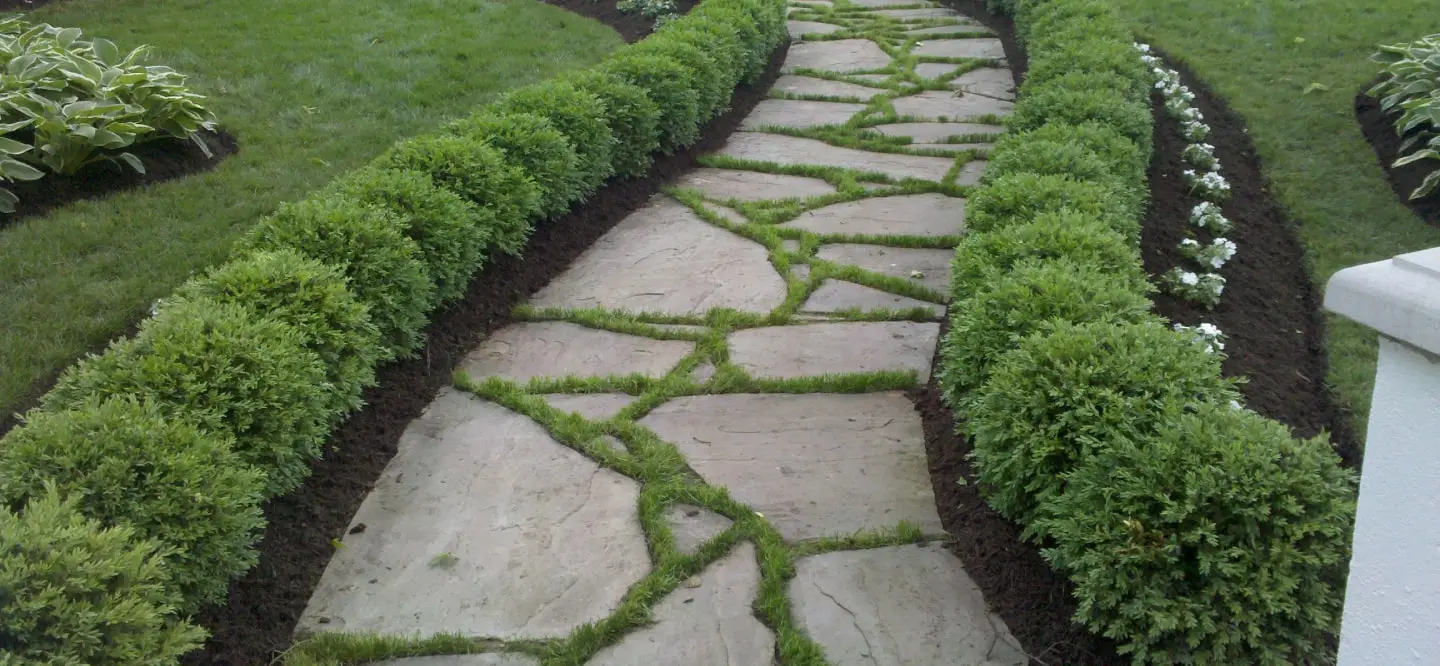# Patio Paver Calculator

Welcome to our Patio Paver Calculator by Stone Center! Planning your dream patio just got easier. Whether you're a DIY enthusiast or a professional landscaper, our user-friendly calculator will help you determine the precise number of pavers and materials needed for your patio project. Say goodbye to guesswork and wasted materials – let our calculator pave the way to your perfect outdoor retreat.

Step 1: Choose The Shape of Area:
Step 2. Fill Out The Data For Each Shape:
Step 3. Sand Base Depth
Step 4: Paver size (in.)
Total Pavers Needed*:
Total Sand Base Needed*:
*Includes standard 10% waste.

## How to Calculate How Many Pavers I Need

Creating a beautiful patio starts with accurate calculations to ensure you have just the right amount of pavers for your project. Follow these simple steps to determine how many pavers you'll need:

### Step 1: Measure the Patio Area

Begin by measuring the length and width of your patio area in feet or meters. Use a tape measure or a measuring wheel to get precise measurements. Record these dimensions as "Length" and "Width."

### Step 2: Calculate the Area

#### Formula for Rectangular or Square Patio

For a rectangular or square patio, calculate the area by multiplying the length and width measurements:

Area (in square feet) = Length (in feet) × Width (in feet)

or

Area (in square meters) = Length (in meters) × Width (in meters)

Make sure your measurements are in the same units (e.g., feet or meters).

#### Formula for a Triangular Patio

For a triangular patio, calculate the area using the formula:

Area (in square feet) = 0.5 × Base (in feet) × Height (in feet)

or

Area (in square meters) = 0.5 × Base (in meters) × Height (in meters)

#### Formula for a Circular Patio

For a circular patio, calculate the area using the formula for the area of a circle:

Area (in square feet) = π × (Radius (in feet))^2

or

Area (in square meters) = π × (Radius (in meters))^2

### Step 3: Account for Waste

To account for cutting and adjustments during installation, it's advisable to add a waste factor. Typically, a waste factor of 5-10% is a good starting point. Multiply your calculated area by the waste factor and add it to your total area:

Total Area with Waste = Area + (Area × Waste Factor)

### Step 4: Choose Paver Size

Select the size and style of pavers you plan to use for your patio. Different pavers come in various sizes and configurations, so choose the one that suits your design preferences.

### Step 5: Calculate Paver Quantity

Divide the total area with waste by the area covered by a single paver. This will give you the approximate number of pavers needed:

Number of Pavers = Total Area with Waste / Area per Paver

### Step 6: Round Up

Pavers are usually sold in whole numbers or by the square foot. Round up the calculated number of pavers to the nearest whole number or the next highest square foot increment, depending on how they are sold.

### Step 7: Double-Check

Before making your final purchase, double-check your calculations and consider ordering a few extra pavers for replacements in the future.

By following these steps, you'll have a precise estimate of how many pavers you need for your patio project, whether it's rectangular, triangular, or circular. Use our Patio Paver Calculator above to streamline this process and get accurate results instantly.

## What If I Have an Irregular Patio Area?

If you have an irregularly shaped patio area, calculating the number of pavers you need may require a bit more effort, but it's still manageable. Here's a brief explanation of how to handle irregular patio areas:

### Calculating Pavers for an Irregular Patio Area

1. Divide into Smaller Sections: Break down your irregular patio area into smaller, more manageable sections. These can be squares, rectangles, or other simpler shapes.
2. Measure Each Section: Measure the length and width of each smaller section individually, just as you would for a regular-shaped area.
3. Calculate Area for Each Section: Calculate the area of each section by multiplying its length by its width.
4. Sum the Areas: Add up the areas of all the individual sections to get the total area of your irregular patio.
5. Account for Waste: As mentioned in the previous steps, apply a waste factor to the total area to allow for cutting and adjustments.
6. Choose Paver Size: Select the paver size you intend to use for your project.
7. Calculate Paver Quantity: Divide the total area with waste by the area covered by a single paver to determine how many pavers you need.
8. Round Up: Round up the calculated number of pavers to the nearest whole number or the next highest square foot increment.

Remember that precision is key when dealing with irregular shapes, so take your time measuring each section accurately. By breaking the irregular area into smaller parts and following these steps, you can still determine the number of pavers needed for your unique patio layout.

## What If I Have Paving Patterns?

When you have specific paving patterns in mind for your irregularly shaped patio area, calculating the number of pavers you need becomes a bit more intricate. Here's a concise guide on how to calculate pavers for irregular patio areas with paving patterns:

### Calculating Pavers for Irregular Patio Areas with Paving Patterns

1. Divide into Pattern Sections: Begin by dividing your irregular patio area into distinct sections based on your chosen paving pattern. These sections may include herringbone, basket weave, running bond, or any other pattern.
2. Measure Each Pattern Section: Measure the length and width of each pattern section separately. Make sure to account for any diagonal or angular measurements that result from the pattern.
3. Calculate Area for Each Pattern Section: Calculate the area of each pattern section by multiplying its length by its width. Keep in mind that for certain patterns like herringbone, you may need to consider the diagonal dimensions.
4. Account for Waste: Apply a waste factor to each pattern section to allow for cutting and adjustments. The waste factor may vary depending on the complexity of the pattern.
5. Choose Paver Size: Select the size and style of pavers you plan to use for your specific pattern.
6. Calculate Paver Quantity for Each Pattern Section: Divide the total area with waste for each pattern section by the area covered by a single paver of your chosen size and style.
7. Round Up for Each Section: Round up the calculated number of pavers for each pattern section to the nearest whole number or the next highest square foot increment, depending on how pavers are sold.
8. Sum the Paver Quantities: Add up the quantities of pavers required for each pattern section to obtain the total number of pavers needed for your entire irregular patio area with the chosen patterns.

### "Running Bond" Pattern Example Calculation

Let's walk through an example of calculating the number of pavers needed for an irregular patio area with a "Running Bond" pattern. In a Running Bond pattern, pavers are laid in rows where each paver is offset by half from the one below it.

Assumptions:

• Irregular patio area dimensions: Length = 20 feet, Width = 15 feet
• Paver size: 6 inches by 6 inches
• Waste factor: 10%
• The Running Bond pattern involves offsetting each row by half a paver's width.

Steps to Calculate Pavers for Running Bond Pattern:

1. Divide into Pattern Sections: For simplicity, we'll consider the entire patio as one pattern section.
2. Measure the Pattern Section: You've measured the area as Length = 20 feet and Width = 15 feet.
3. Calculate Area for the Pattern Section:
Area (in square feet) = Length (in feet) × Width (in feet) Area = 20 feet × 15 feet = 300 square feet
4. Account for Waste: Apply a 10% waste factor to account for cutting and adjustments:
Total Area with Waste = Area + (Area × Waste Factor) Total Area with Waste = 300 square feet + (300 square feet × 0.10) = 330 square feet
5. Choose Paver Size: The paver size is 6 inches by 6 inches, which is 0.5 feet by 0.5 feet.
6. Calculate Paver Quantity for the Pattern Section: Divide the total area with waste by the area covered by a single paver:
Number of Pavers = Total Area with Waste / Area per Paver Number of Pavers = 330 square feet / (0.5 feet × 0.5 feet) = 1320 pavers
7. Round Up: Since pavers are typically sold in whole numbers or by square foot increments, round up the calculated number of pavers to the nearest whole number:
Rounded-Up Number of Pavers = 1320 pavers (rounded up to the nearest whole number)

So, for a Running Bond pattern on your irregular patio area, you’d need approximately 1,320 pavers. This calculation accounts for both the pattern and the waste factor to ensure you have enough pavers to complete your project.

## Common Paver Patterns

Pavers offer a wide range of design possibilities, and different paver patterns can create distinct looks for your patio, driveway, or walkway. We’ve outlined some common paver patterns used in landscaping and hardscaping projects:

1. Running Bond (Brick Bond):
• Pavers are laid in rows, with each paver in a row offset by half from the one below it.
• This pattern resembles the way bricks are often laid in walls.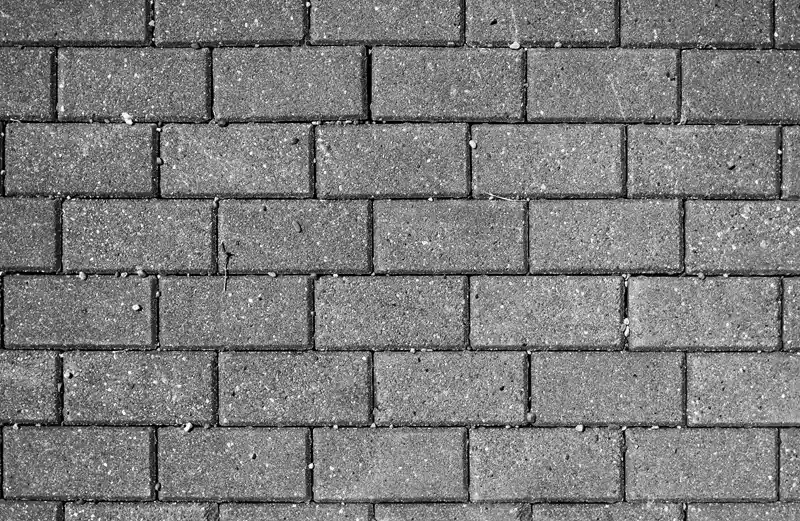2. Herringbone:
• Pavers are set at a 45-degree or 90-degree angle to create a zigzag or "V" pattern.
• Provides a strong and visually appealing design.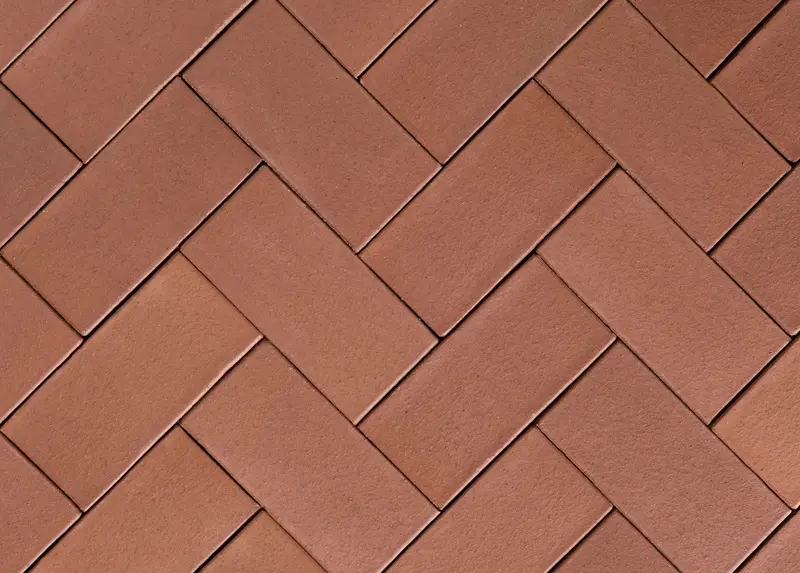• Pavers are arranged in pairs, with each pair consisting of two perpendicular pavers.
• Creates a woven or basket-like appearance.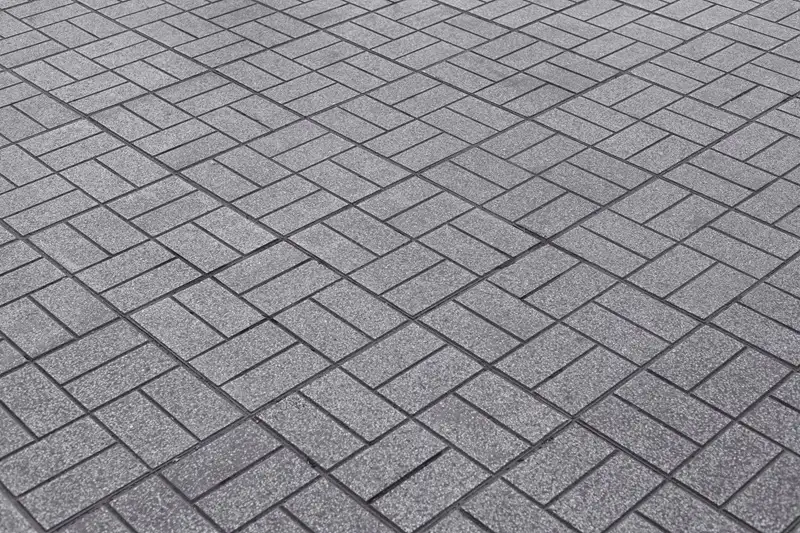4. Stack Bond:
• Pavers are stacked directly on top of each other in a grid pattern.
• This pattern is simple and has a contemporary style.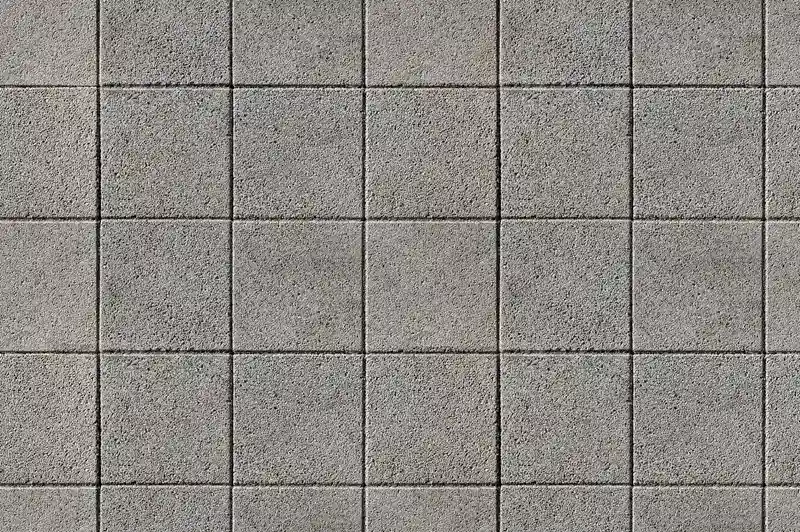5. Circular:
• Pavers are laid in a circular or radial pattern.
• Ideal for creating focal points or circular patios.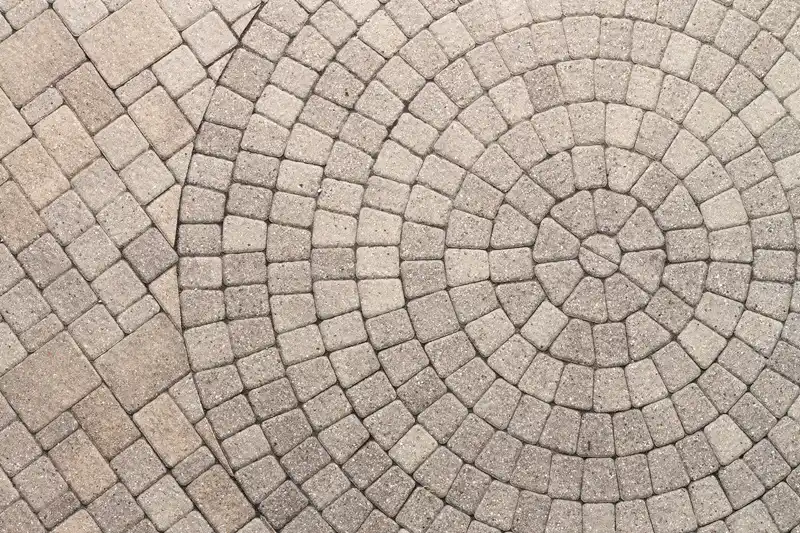• Pavers are arranged in a fan or wedge shape, radiating out from a central point.
• Adds a dynamic, decorative element to the design.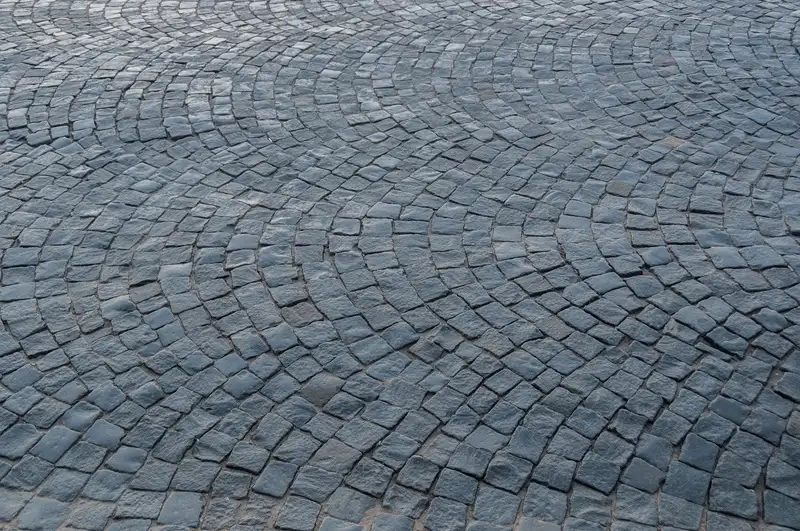7. Mosaic:
• Pavers of various sizes and shapes are combined to create intricate, mosaic-like patterns.
• Allows for artistic and creative designs.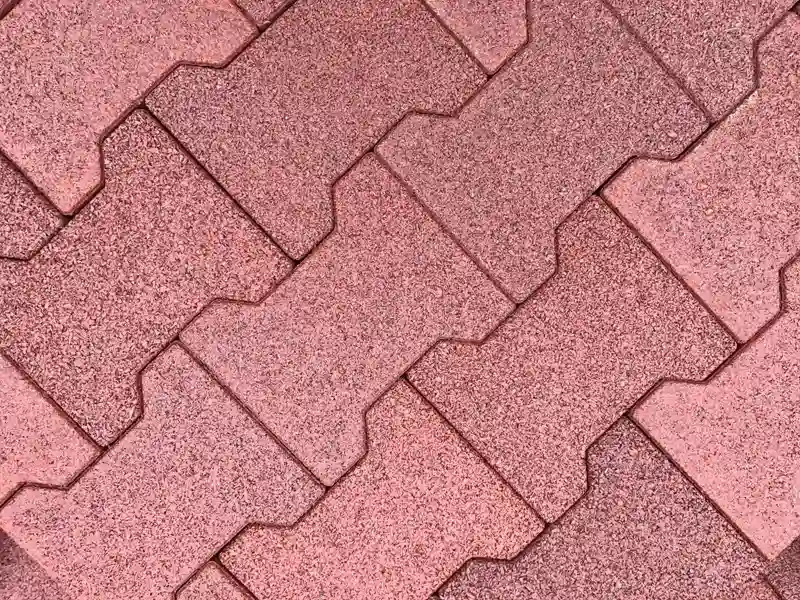8. Grid:
• Pavers are set in a grid formation, with equal spacing between each paver.
• Offers a clean and modern aesthetic.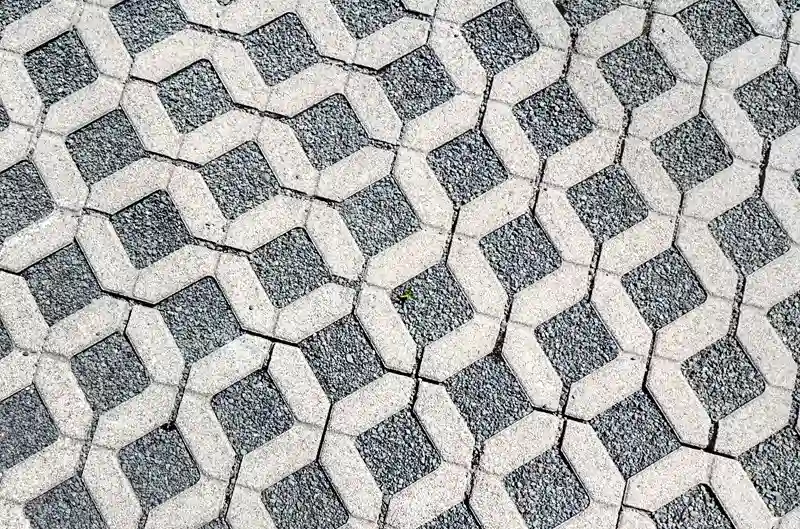9. Dutch Pattern:
• Also known as a "5-size" pattern, it uses multiple paver sizes in a predetermined arrangement.
• Creates visual interest and complexity.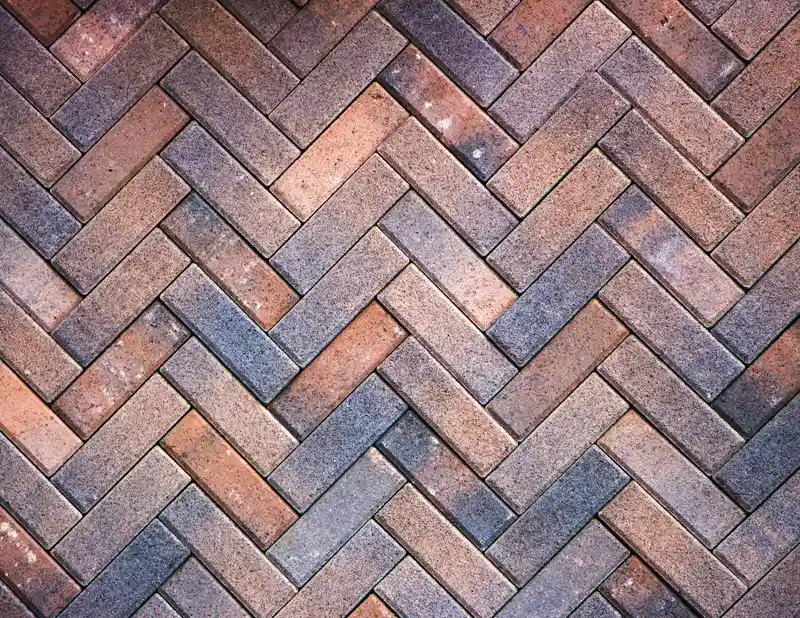10. Circle in Square:
• A circular pattern is surrounded by a square border.
• Creates a well-defined center point with a structured outline.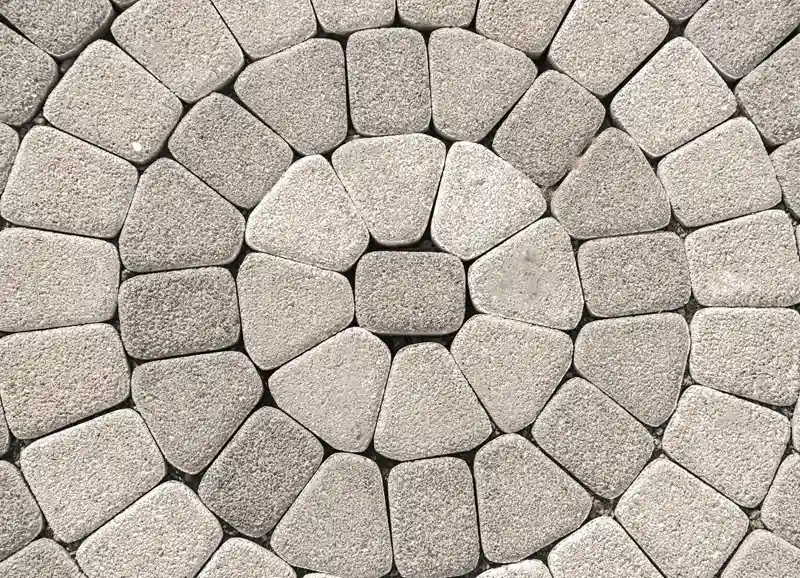11. Hexagonal:
• Pavers are laid in a hexagonal, honeycomb-like pattern.
• Offers a unique geometric design.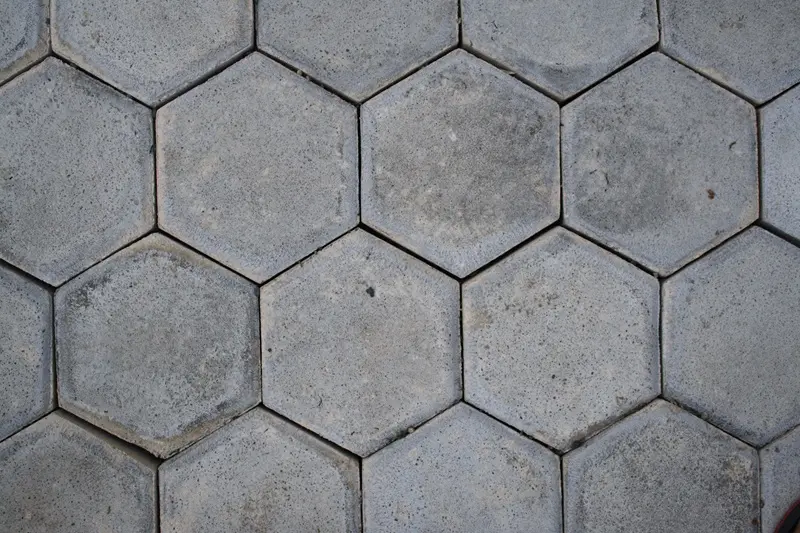12. Offset Running Bond:
• Similar to Running Bond, but with the pavers in each row staggered by a fraction of their length.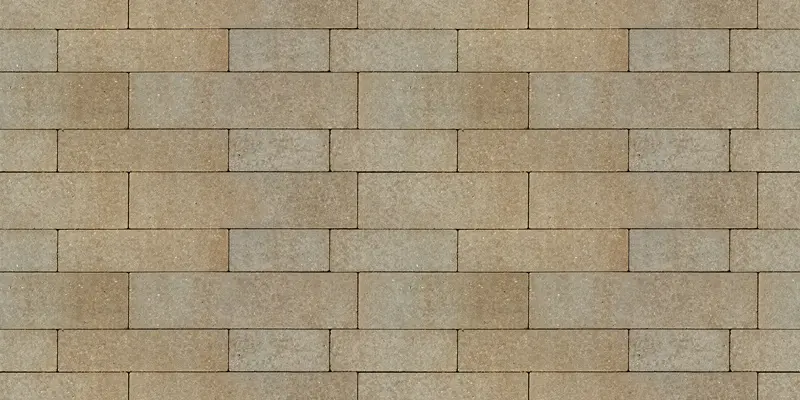Choosing the right paver pattern depends on your aesthetic preferences, the layout of your outdoor space, and the overall design goals of your project. Each pattern can lend a distinct character and visual appeal to your hardscape.

## Common Paver Sizes

Here's a table showcasing common paver sizes, along with their dimensions and common applications:

Paver Size Dimensions (centimeters) Coverage (square feet) Common Applications
4" x 8" 10 cm x 20 cm 0.22 sq. ft. Walkways, borders, accent pieces
6" x 6" 15 cm x 15 cm 0.25 sq. ft. Patios, small walkways
6" x 9" 15 cm x 23 cm 0.375 sq. ft. Patios, small driveways
8" x 8" 20 cm x 20 cm 0.44 sq. ft. Patios, courtyards, decorative
12" x 12" 30 cm x 30 cm 1 sq. ft. Large patios, pathways, pool decks
12" x 24" 30 cm x 61 cm 2 sq. ft. Driveways, larger patio areas
24" x 24" 61 cm x 61 cm 4 sq. ft. Large, contemporary designs